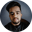Related Tags

c#
communitycreator

# How to generate random numbers in C#Shubham Singh Kshatriya

### Overview

The C# language provides a built-in class, Random, for generating random numbers based on a seed value. By default, the Random class uses a system clock to generate a seed value.

The Random class provides the following methods to generate random numbers.

### 1. The Next() method

This method generates a random positive number within the range -2,147,483,648 to 2,147,483,647.

#### Syntax

Random random = new Random();
int num = random.Next();
Syntax of the Next() method

#### Example

Let's see an example of the Next() method in the code snippet below:

using System;

class Example
{
static void Main()
{
// instantiate Random class
Random random = new Random();

// generate a random number
int n = random.Next();

// print the number of console
Console.WriteLine("Random number: {0}", n);
}
}
Example of the Next() method

#### Explanation

Inside the main() function:

• Line 8: We instantiate the Random class and create an object called random.
• Line 11: We generate a random positive number using the Next() method.
• Line 14: We print the random number on the console.

### 2. The Next(int) method

This method generates a random number that is less than the specified integer. Here, the specified integer acts as the upper bound.

#### Syntax

Random random = new Random();
int num = random.Next(int);
Syntax of the Next(int) method

#### Example

Let's see an example of the Next(int) method in the code snippet below:

using System;

class Example
{
static void Main()
{
// instantiate Random class
Random random = new Random();

// generate a random number
int n = random.Next(50);

// print the number of console
Console.WriteLine("Random number: {0}", n);
}
}
Example of the Next(int) method

#### Explanation

Inside the main() function:

• Line 8: We instantiate the Random class and create an object called random.
• Line 11: We generate a random number using the Next(int) method.
• Line 14: We print the random number on the console.

### 3. The Next(int, int) method

This method generates a random number within the specified range. Here, the first integer acts as the lower bound and the second integer acts as the upper bound.

#### Syntax

Random random = new Random();
int num = random.Next(int, int);
Syntax of the Next(int, int) method

#### Example

Let's see an example of the Next(int, int) method in the code snippet below:

using System;

class Example
{
static void Main()
{
// instantiate Random class
Random random = new Random();

// generate a random number
int n = random.Next(20, 30);

// print the number of console
Console.WriteLine("Random number: {0}", n);
}
}
Example of the Next(int, int) method

#### Explanation

Inside the main() function:

• Line 8: We instantiate the Random class and create an object called random.
• Line 11: We generate a random number using the Next(int, int) method in the range (20, 30).
• Line 14: We print the random number on the console.

### 4. The NextDouble() method

This method generates a random floating number within the range (0.0, 1.0).

#### Syntax

Random random = new Random();
int num = random.NextDouble();
Syntax of the NextDouble() method

#### Example

Let's see an example of the NextDouble() method in the code snippet below:

using System;

class Example
{
static void Main()
{
// instantiate Random class
Random random = new Random();

// generate a random number
double n = random.NextDouble();

// print the number of console
Console.WriteLine("Random number: {0}", n);
}
}
Example of the NextDouble() method

#### Explanation

Inside the main() function:

• Line 8: We instantiate the Random class and create an object called random.
• Line 11: We generate a random floating number using the NextDouble() method.
• Line 14: We print the random number on the console.

RELATED TAGS

c#
communitycreator

CONTRIBUTORShubham Singh Kshatriya
RELATED COURSES

View all Courses

Keep Exploring

Learn in-demand tech skills in half the time